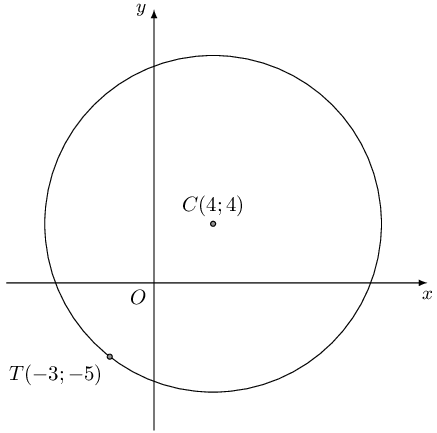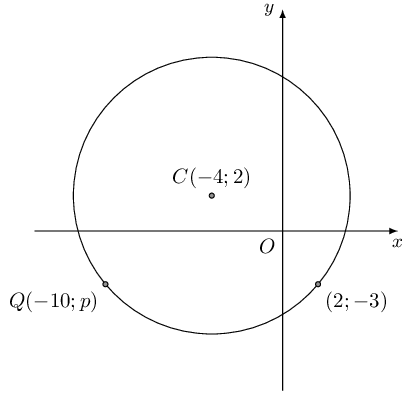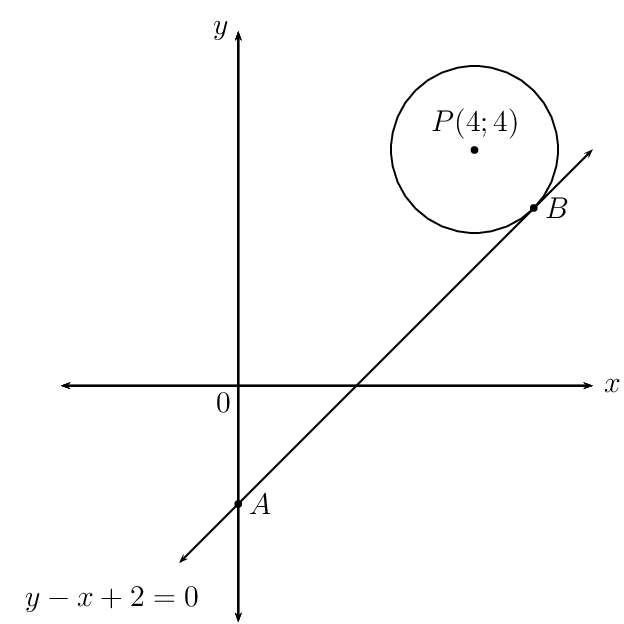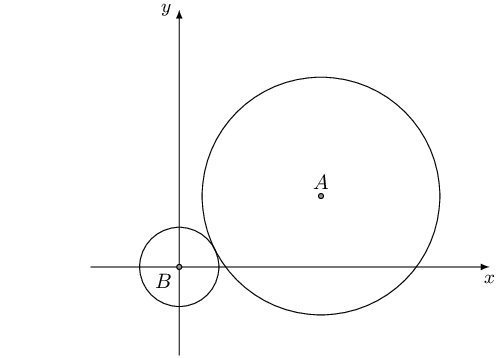Home Practice
For learners and parents For teachers and schools
Textbooks
Full catalogue
Pricing SupportLog in

We think you are located in United States. Is this correct?

# End of chapter exercises

## End of chapter exercises

Textbook Exercise 7.6

Find the equation of the circle:

with centre $$\left(0;5\right)$$ and radius $$\text{5}$$

\begin{align*} (x)^2 + (y-5)^2 &= 5^{2} \\ (x)^2 + (y-5)^2 &= 25 \\ & \\ \text{Expanded: } x^2 + y^2 -10y + 25 &= 25 \\ x^2 + y^2 - 10y &=0 \end{align*}

with centre $$\left(2;0\right)$$ and radius $$\text{4}$$

\begin{align*} (x-2)^2 + y^2 &= 16 \\ & \\ \text{Expanded: } x^2 -4x + 4 + y^2 &= 16 \\ x^2 - 4x + y^2 - 12 &= 0 \end{align*}

with centre $$\left(-5;7\right)$$ and radius $$\text{18}$$

\begin{align*} (x+5)^2 + (y-7)^2 &= 18^{2} \\ (x+5)^2 + (y-7)^2 &= 324\\ & \\ \text{Expanded: } x^2 + 10x + 25 + y^2 -14y + 49 &= 324 \\ x^2 + 10x + y^2 -14y - 250 &=0 \end{align*}

with centre $$\left(-2;0\right)$$ and diameter $$\text{6}$$

\begin{align*} (x+2)^2 + y^2 &= 9 \\ & \\ \text{Expanded: } x^2 + 4x + 4 + y^2 - 9 &= 0 \\ x^2 + 4x + y^2 - 5 &= 0 \end{align*}

with centre $$\left(-5;-3\right)$$ and radius $$\sqrt{3}$$

\begin{align*} (x+5)^2 + (y+3)^2 &= 3 \\ & \\ \text{Expanded: } x^2 + 10x + 25 + y^2 + 6y + 9 &= 3 \\ x^2 + 10x + y^2 + 6y + 31 &= 0 \end{align*}

Find the equation of the circle with centre $$\left(2;1\right)$$ which passes through $$\left(4;1\right)$$.

\begin{align*} (x - 2)^2 + (y - 1)^2 &= r^{2} \\ (4 - 2)^2 + (1 - 1)^2 &= r^{2} \\ (2)^2 + (0)^2 &= r^{2} \\ 4 &= r^{2} \\ \therefore r &= 2 \\ (x - 2)^2 + (y - 1)^2 &= 4 \\ & \\ \text{Expanded: } x^2 - 4x + y^2 - 2y + 1 &= 0 \end{align*}

Where does it cut the line $$y=x+1$$?

\begin{align*} (x - 2)^2 + (y - 1)^2 &= 4 \\ (x - 2)^2 + (x + 1 - 1)^2 &= 4 \\ (x - 2)^2 + (x)^2 &= 4 \\ x^2 - 4x + 4 + x^2 &= 4 \\ 2x^2 - 4x &= 0 \\ x^2 - 2x &= 0 \\ x(x - 2) &= 0 \\ \therefore x = 0 &\text{ or } x = 2 \\ \text{If } x = 0, y &= 1 \quad (0;1) \\ \text{If } x = 2, y &= 3 \quad (2;3) \end{align*}

Find the equation of the circle with centre $$\left(-3;-2\right)$$ which passes through $$\left(1;-4\right)$$.

\begin{align*} (x + 3)^2 + (y + 2)^2 &= r^{2} \\ (1 + 3)^2 + (-4 + 2)^2 &= r^{2} \\ (4)^2 + (-2)^2 &= r^{2} \\ 16 + 4 &= r^{2} \\ 20 &= r^{2} \\ (x + 3)^2 + (y + 2)^2 &= 20 \end{align*}

Find the equation of the circle with centre $$\left(3;1\right)$$ which passes through $$\left(2;5\right)$$.

\begin{align*} (x - 3)^2 + (y - 1)^2 &= r^{2} \\ (2 - 3)^2 + (5 - 1)^2 &= r^{2} \\ (-1)^2 + (4)^2 &= r^{2} \\ 1 + 16 &= r^{2} \\ 17&= r^{2} \\ (x - 3)^2 + (y - 1)^2 &= 17 \end{align*}

Find the centre and radius of the following circles:

$${\left(x+9\right)}^{2}+{\left(y-6\right)}^{2}=36$$

$$(-9;6)$$, $$r = 6$$ units

$$\frac{1}{2}{\left(x-2\right)}^{2}+\frac{1}{2}{\left(y-9\right)}^{2}=1$$

$$(2;9)$$, $$r = \sqrt{2}$$ unit

$${\left(x+5\right)}^{2}+{\left(y+7\right)}^{2}=12$$

$$(-5;-7)$$, $$r = \sqrt{12}$$ units

$$x^{2}+{\left(y+4\right)}^{2}=23$$

$$(0;-4)$$, $$r = \sqrt{23}$$ units

$$3{\left(x-2\right)}^{2}+3{\left(y+3\right)}^{2}=12$$

$$(2;-3)$$, $$r = 2$$ units

Find the $$x$$ and $$y$$ intercepts of the following graphs:

$${x}^{2}+{\left(y-6\right)}^{2}=100$$

\begin{align*} {x}^{2}+{\left(y-6\right)}^{2}&=100 \\ \text{Let } x = 0: \quad {\left(y-6\right)}^{2}&=100 \\ y^{2} - 12y + 36 &= 100 \\ y^{2} - 12y - 64 &= 0 \\ (y - 16)(y + 4) &= 0 \\ \therefore y = 16 &\text{ or } y = -4 \\ (0;16) &\text{ and } (0;-4)\\ & \\ {x}^{2}+{\left(y-6\right)}^{2}&=100 \\ \text{Let } y = 0: \quad {x}^{2}+{\left(-6\right)}^{2}&=100 \\ {x}^{2}+ 36 &=100 \\ {x}^{2} &= 64 \\ \therefore x = -8 &\text{ or } x = 8 \\ (-8;0) &\text{ and } (8;0) \end{align*}

$${\left(x+4\right)}^{2}+{y}^{2}=16$$

\begin{align*} {\left(x+4\right)}^{2}+{y}^{2} &= 16 \\ \text{Let } x = 0: \quad {\left(x+4\right)}^{2}+{y}^{2} &= 16 \\ 4^{2} + y^{2} &= 16 \\ y^{2} &= 0 \\ \therefore & (0;0) \\ & \\ {\left(x+4\right)}^{2}+{y}^{2} &= 16 \\ \text{Let } y = 0: \quad ({x} + 4)^{2}&=16 \\ x^{2} + 8x + 16 &=16 \\ x^{2} + 8x &= 0 \\ x(x + 8) &= 0 \\ \therefore x = 0 &\text{ or } x = -8 \\ (0;0) &\text{ and } (-8;0) \end{align*}

Find the centre and radius of the following circles:

$${x}^{2}+6x+{y}^{2}-12y=-20$$

\begin{align*} {x}^{2}+6x+{y}^{2}-12y &= -20 \\ ({x} + 3)^{2} - 9 + ({y} - 6)^{2} - 36 &= -20 \\ ({x} + 3)^{2} + ({y} - 6)^{2} &= 25 \end{align*}

The centre of the circle is $$(-3;6)$$ and $$r = 5$$ units.

$${x}^{2}+4x+{y}^{2}-8y=0$$

\begin{align*} {x}^{2}+4x+{y}^{2}-8y &= 0 \\ ({x} + 2)^{2} - 4 + ({y} - 4)^{2} - 16 &= 0 \\ ({x} + 2)^{2} + ({y} - 4)^{2} &= 20 \end{align*}

The centre of the circle is $$(-2;4)$$ and $$r = \sqrt{20}$$ units.

$${x}^{2}+{y}^{2}+8y=7$$

\begin{align*} {x}^{2}+{y}^{2}+8y &= 7 \\ {x}^{2} + ({y} + 4)^{2} - 16 &= 7 \\ {x}^{2} + ({y} + 4)^{2} &= 23 \end{align*}

The centre of the circle is $$(0;-4)$$ and $$r = \sqrt{23}$$ units.

$${x}^{2}-6x+{y}^{2}=16$$

\begin{align*} {x}^{2}-6x+{y}^{2} &= 16 \\ (x - 3)^{2} - 9 + y^{2} &= 16 \\ (x - 3)^{2} + y^{2} &= 25 \end{align*}

The centre of the circle is $$(3;0)$$ and $$r = 5$$ units.

$${x}^{2}-5x+{y}^{2}+3y=-\frac{3}{4}$$

\begin{align*} {x}^{2}-5x+{y}^{2}+3y &= -\frac{3}{4} \\ \left(x - \frac{5}{2}\right)^{2} - \frac{25}{4} + \left(y + \frac{3}{2}\right)^{2} - \frac{9}{4} &= -\frac{3}{4} \\ \left(x - \frac{5}{2}\right)^{2} + \left(y + \frac{3}{2}\right)^{2} &= \frac{31}{4} \end{align*}

The centre of the circle is $$(\frac{5}{2};-\frac{3}{2})$$ and $$r = \frac{\sqrt{31}}{2}$$ units.

$${x}^{2}-6nx+{y}^{2}+10ny=9{n}^{2}$$

\begin{align*} {x}^{2}-6nx+{y}^{2}+10ny &= 9{n}^{2} \\ (x - 3n)^{2} - 9n^{2} + (y + 5n)^{2} - 25n^{2} &= 9{n}^{2} \\ (x - 3n)^{2} + (y + 5n)^{2} &= 43{n}^{2} \end{align*}

The centre of the circle is $$(3n;-5n)$$ and $$r = \sqrt{43}n$$ units.

Find the gradient of the radius between the point $$(4;5)$$ on the circle and its centre $$(-8;4)$$.

Given:

• the centre of the circle $$(a;b) = (-8;4)$$
• a point on the circumference of the circle $$(4;5)$$

Required:

• the gradient $$m$$ of the radius
\begin{align*} m & = \frac{y_2 - y_1}{x_2 - x_1}\\ & = \frac{5-4}{4+8}\\ & = \frac{1}{12} \end{align*}

The gradient for this radius is $$m = \frac{1}{12}$$.

Find the gradient line tangent to the circle at the point $$(4;5)$$.

The tangent to the circle at the point $$(4;5)$$ is perpendicular to the radius of the circle to that same point:

\begin{align*} m_{\bot} & = - \frac{1}{m}\\ & = \frac{-1}{\frac{1}{12} }\\ & = -12 \end{align*}

The gradient for the tangent is $$m_{\bot} = -12$$.

Given $$\left(x - 1\right)^{2} + \left(y - 7\right)^{2} = 10$$, determine the value(s) of $$x$$ if $$(x;4)$$ lies on the circle.

\begin{align*} (x - 1)^{2} + (4 - 7)^{2} &= 10 \\ x^{2} - 2x + 1 + 9 &= 10 \\ x(x - 2) &= 0 \\ \therefore x = 0 &\text{ or } x = 2 \end{align*}

The points $$(0;4)$$ and $$(2;4)$$ lie on the circle.

$$(0;4),(2;4)$$

Find the gradient of the tangent to the circle at the point $$(2;4)$$.

\begin{align*} m_r & = \frac{y_1 - y_0}{x_1 - x_0} \\ & = \frac{4 - 7}{2 - 1} \\ & = -3 \end{align*} \begin{align*} m_{\text{tangent}} & = - \frac{1}{m}\\ & = - \dfrac{1}{-3}\\ \\ & = \frac{1}{3} \end{align*}

The gradient of the tangent is $$m_{\text{tangent}} = \frac{1}{3}$$.

$$m = \frac{1}{3}$$

Given a circle with the central coordinates $$(a;b) = (-2;-2)$$. Determine the equation of the tangent line of the circle at the point $$(-1;3)$$.

\begin{align*} m_r & = \frac{y_1 - y_0}{x_1 - x_0} \\ & = \frac{3 - (-2)}{-1 -(-2)} \\ & = 5 \end{align*}

The radius is perpendicular to the tangent, therefore $$m_{r} \times m_{\bot} = -1$$:

$m_{\bot} = - \frac{1}{5}$

Substitute $$m = - \frac{1}{5}$$ and $$(-1;3)$$ to determine $$c$$:

\begin{align*} y & =m_{\bot} x + c\\ 3 & = - \frac{1}{5} (-1) + c \\ c & = \frac{14}{5} \end{align*}

The equation of the tangent to the circle at the point $$(-1;3)$$ is $$y = - \frac{1}{5} x + \frac{14}{5}$$.

$$y = - \frac{1}{5} x + \frac{14}{5}$$

Consider the diagram below:Find the equation of the tangent to the circle at point $$T$$.

\begin{align*} m_r & = \frac{y_{1} - y_0}{x_{1} - x_0} \\ & = \frac{4+5}{4+3 } \\ & = \frac{9}{7} \end{align*} \begin{align*} m_r \times m & = -1 \\ \therefore m & = - \frac{1}{m_r} \\ & = - \dfrac{1}{\frac{9}{7} } \\ & = - \frac{7}{9} \end{align*}

Determine the $$y$$-intercept ($$c$$) of the line by substituting the point $$T(-3;-5)$$.

\begin{align*} y & = m x + c\\ -5 & = - \frac{7}{9} (-3) + c \\ c & = - \frac{22}{3} \end{align*}

The equation of the tangent to the circle at $$T$$ is

\begin{align*} y & = - \frac{7}{9} x - \frac{22}{3} \end{align*} $$y = - \frac{7}{9} x - \frac{22}{3}$$

$$M(-2;-5)$$ is a point on the circle $$x^{2} + y^{2} + 18y + 61 = 0$$. Determine the equation of the tangent at $$M$$.

Complete the square:

\begin{align*} x^{2} + y^{2} + 18y + 61 &= 0 \\ x^{2} + (y^{2} + 18y) &= -61 \\ x^{2} + (y + 9)^{2} - 81 &= -61 \\ x^{2} + (y + 9)^{2} &= 20 \end{align*}

Therefore the centre of the circle is $$(0;-9)$$ and $$r = \sqrt{20}$$ units.

\begin{align*} m_r & = \frac{y_1 - y_0}{x_1 - x_0} \\ & = \frac{-5 - (-9)}{-2 - 0} \\ & = \frac{4}{-2} \\ & = -2 \end{align*} \begin{align*} m_{\bot} & = - \frac{1}{m_r} \\ & = - \frac{1}{-2} \\ & = \frac{1}{2} \end{align*}

Determine the $$y$$-intercept $$c$$ of the line by substituting the point $$M(-2;-5)$$.

\begin{align*} y & = m_{\bot} x + c\\ -5 & = \frac{1}{2} (-2) + c \\ c & = -4 \end{align*}

The equation for the tangent to the circle at the point $$M(-2;-5)$$ is

\begin{align*} y & = \frac{1}{2} x - 4 \end{align*} $$y = \frac{1}{2} x - 4$$

$$C(-4;2)$$ is the centre of the circle passing through $$(2;-3)$$ and $$Q(-10;p)$$.Find the equation of the circle given.

\begin{align*} r & = \sqrt{(x_1 - x_0)^2 + (y_1 - y_0)^2} \\ & = \sqrt{(2+4)^2 + (-3-2)^2} \\ & = \sqrt{(6)^2 + (-5)^2} \\ & = \sqrt{61} \end{align*} \begin{align*} (x-a)^2+ (y-b)^2 & = r^2 \\ (x - (-4))^2 + (y-(2))^2 & = (\sqrt{61})^2 \\ \left(x + 4\right)^{2} + \left(y - 2\right)^{2} & = 61 \end{align*}

The equation of the circle is $$\left(x + 4\right)^{2} + \left(y - 2\right)^{2} = 61$$.

$$\left(x + 4\right)^{2} + \left(y - 2\right)^{2} = 61$$

Determine the value of $$p$$.

\begin{align*} \left(x + 4\right)^{2} + \left(y - 2\right)^{2} & = 61 \\ \left(-10 + 4\right)^{2} + \left(p - 2\right)^{2} & = 61 \\ \left(-10 + 4\right)^{2} + p^{2} - 4p + 4 & = 61 \\ 36 + p^{2} - 4p + 4 & = 61 \\ p^{2} - 4p - 21 & = 0 \\ (p+3)(p-7) & = 0 \\ \therefore p = -3 & \text{ or } p = 7 \end{align*}

From the graph we see that the correct $$y$$-value is $$-\text{3}$$.

The coordinates for point $$Q$$ is $$Q(-10;-3)$$

$$p = -3$$

Determine the equation of the tangent to the circle at point $$Q$$.

\begin{align*} m_r & = \frac{y_2 - y_0}{x_2 - x_0} \\ & = \frac{-3 -2}{-10 -(-4) } \\ & = \frac{5}{6} \end{align*}

$$m_{r} \times m_{\bot} = -1$$.

\begin{align*} m_{\bot} & = - \frac{1}{m_r} \\ & = - \dfrac{1}{\frac{5}{6}} \\ & = - \frac{6}{5} \end{align*}

Determine the $$y$$-intercept $$c$$ of the line by substituting the point $$Q(x_2;y_2) = (-10;-3)$$.

\begin{align*} y_2 & = m_{\bot} x_2 + c\\ -3 & = - \frac{6}{5} (-10) + c \\ c & = -15 \end{align*}

The equation of the tangent to the circle at $$Q$$ is $$y = - \frac{6}{5} x - 15$$.

Find the equation of the tangent to each circle:

$${x}^{2}+{y}^{2}=17$$ at the point $$\left(1;4\right)$$

The centre of the circle is $$(0;0)$$ and $$r = \sqrt{17}$$ units.

\begin{align*} m_{r} & = \frac{y_2 - y_1}{x_2 - x_1} \\ & = \frac{4 - 0}{1 - 0} \\ & = 4 \end{align*} \begin{align*} m_{r} & \times m_{\bot} = -1 \\ m_{\bot} & = - \frac{1}{m_{r}} \\ & = - \dfrac{1}{4} \\ & = - \frac{1}{4} \end{align*} \begin{align*} y_2 & = m_{\bot} x_2 + c\\ 4 & = - \frac{1}{4}(1) + c \\ c & = \frac{17}{4} \end{align*}

The equation of the tangent to the circle is $$y = - \frac{1}{4} x + \frac{17}{4}$$.

$${x}^{2}+{y}^{2}=25$$ at the point $$\left(3;4\right)$$

The centre of the circle is $$(0;0)$$ and $$r = 5$$ units.

\begin{align*} m_{r} & = \frac{y_2 - y_1}{x_2 - x_1} \\ & = \frac{4 - 0}{3 - 0} \\ & = \frac{4}{3} \end{align*} \begin{align*} m_{r} & \times m_{\bot} = -1 \\ m_{\bot} & = - \frac{1}{m_{r}} \\ & = - \dfrac{1}{\frac{4}{3} } \\ & = - \frac{3}{4} \end{align*} \begin{align*} y_2 & = m_{\bot} x_2 + c\\ 4 & = - \frac{3}{4}(3) + c \\ c & = \frac{25}{4} \end{align*}

The equation of the tangent to the circle is $$y = - \frac{3}{4} x + \frac{25}{4}$$.

$${\left(x+1\right)}^{2}+{\left(y-2\right)}^{2}=25$$ at the point $$\left(3;5\right)$$

The centre of the circle is $$(-1;2)$$ and $$r = 5$$ units.

\begin{align*} m_{r} & = \frac{y_2 - y_1}{x_2 - x_1} \\ & = \frac{5-2}{3 - (-1)} \\ & = \frac{3}{4} \end{align*} \begin{align*} m_{r} & \times m_{\bot} = -1 \\ m_{\bot} & = - \frac{1}{m_{r}} \\ & = - \dfrac{1}{\frac{3}{4} } \\ & = - \frac{4}{3} \end{align*} \begin{align*} y_2 & = m_{\bot} x_2 + c\\ 5 & = - \frac{4}{3}(3) + c \\ c & = 9 \end{align*}

The equation of the tangent to the circle is $$y = - \frac{4}{3} x + 9$$.

$${\left(x-2\right)}^{2}+{\left(y-1\right)}^{2}=13$$ at the point $$\left(5;3\right)$$

The centre of the circle is $$(2;1)$$ and $$r = \sqrt{13}$$ units.

\begin{align*} m_{r} & = \frac{y_2 - y_1}{x_2 - x_1} \\ & = \frac{3-1}{5-2} \\ & = \frac{2}{3} \end{align*} \begin{align*} m_{r} & \times m_{\bot} = -1 \\ m_{\bot} & = - \frac{1}{m_{r}} \\ & = - \dfrac{1}{\frac{2}{3} } \\ & = - \frac{3}{2} \end{align*} \begin{align*} y_2 & = m_{\bot} x_2 + c\\ 3 & = - \frac{3}{2}(5) + c \\ c & = \frac{21}{2} \end{align*}

The equation of the tangent to the circle is $$y = - \frac{3}{2} x + \frac{21}{2}$$.

Determine the equations of the tangents to the circle $$x^{2} + y^{2} = 50$$, given that both lines have an angle of inclination of $$\text{45} °$$.

The centre of the circle is $$(0;0)$$ and $$r = \sqrt{50}$$ units.

\begin{align*} m & = \tan \theta \\ & = \tan{ \text{45} °} \\ & = 1 \end{align*} \begin{align*} m & \times m_{\bot} = -1 \\ m & = - 1 \end{align*}

The line perpendicular to the tangents and passing through the centre of the circle is $$y = -x$$. Substitute $$y = -x$$ into the equation of the circle and solve for $$x$$:

\begin{align*} x^{2} + (-x)^{2} &= 50 \\ 2x^{2} &= 50 \\ x^{2} &= 25 \\ x &= \pm 5 \end{align*}

This gives the points $$P(-5;5)$$ and $$Q(5;-5)$$.

Tangent at $$P(-5;5)$$:

\begin{align*} y - 5 & = (1)(x - (-5)) \\ y & = x + 10 \end{align*}

Tangent at $$Q(5;-5)$$:

\begin{align*} y - (-5) & = (1)(x - 5) \\ y & = x - 10 \end{align*}

The equations of the tangents to the circle are $$y = x - 10$$ and $$y = x + 10$$.

The circle with centre $$P(4; 4)$$ has a tangent $$AB$$ at point $$B$$. The equation of $$AB$$ is $$y - x + 2 = 0$$ and $$A$$ lies on the $$y$$-axis.Determine the equation of $$PB$$.

\begin{align*} m_{AB} & = 1 \\ \therefore m_{PB} & = - 1 \\ y & = mx + c \\ \text{Substitute } P(4;4): \quad 4 & = -(4) + c \\ \therefore c &= 8 \\ y &= -x + 8 \end{align*} $$y = -x + 8$$

Determine the coordinates of $$B$$.

Equate the two equations and solve for $$x$$:

\begin{align*} x -2 & = -x + 8 \\ 2x & = 10 \\ x & = 5 \\ y &= -5 + 8 \\ \therefore y &= 3 \end{align*}

$$B(5;3)$$

Determine the equation of the circle.

\begin{align*} PB^{2} & = (5 -4)^{2} + (3 - 4)^{2} \\ & = 1 + 1 \\ & = 2 \\ (x -4)^{2} + (y - 4)^{2} & = 2 \end{align*} $$(x -4)^{2} + (y - 4)^{2} = 2$$

Describe in words how the circle must be shifted so that $$P$$ is at the origin.

The circle must be shifted $$\text{4}$$ units down and $$\text{4}$$ units to the left.

If the length of $$PB$$ is tripled and the circle is shifted $$\text{2}$$ units to the right and $$\text{1}$$ unit up, determine the equation of the new circle.

\begin{align*} PB & = \sqrt{2} \\ 3 \times PB & = 3\sqrt{2} \\ \text{Horizontal shift: } (x -4 - 2)^{2} + (y - 4)^{2} & = \left( 3\sqrt{2} \right)^2 \\ (x -6)^{2} + (y - 4)^{2} & = 9(2) \\ \text{Vertical shift: } (x -6)^{2} + (y - 4 -1)^{2} & = 18 \\ (x -6)^{2} + (y - 5)^{2} & = 18 \end{align*}

The equation of a circle with centre $$A$$ is $$x^{2} + y^{2} + 5 = 16x + 8y - 30$$ and the equation of a circle with centre $$B$$ is $$5x^{2} + 5y^{2} = 25$$. Prove that the two circles touch each other.

\begin{align*} x^{2} + y^{2} + 5 & = 16x + 8y - 30 \\ x^{2} -16x + y^{2} - 8y & = - 35 \\ (x - 8)^{2} -64 + (y - 4)^{2} -16 & = - 35 \\ (x - 8)^{2} + (y - 4)^{2} & = 45 \\ & \\ 5x^{2} + 5y^{2} &= 25 \\ x^{2} + y^{2} &= 5 \\ & \\ AB^{2} &= (8 - 0)^{2} + (4 - 0)^{2} \\ &= 64 + 16 \\ &= 80\\ \therefore AB &= \sqrt{80} \\ AB &= 4\sqrt{5} \\ & \\ \text{And } \text{radius}_{A} + \text{radius}_{B} &= \sqrt{45} + \sqrt{5} \\ &= 3\sqrt{5} + \sqrt{5} \\ &= 4\sqrt{5} \\ &=AB \end{align*}

Therefore the two circles touch each other.